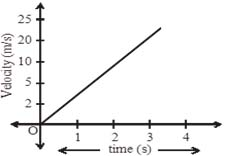# ICSE Worksheet for chapter-5 Motion in One Dimension class 9

## Find ICSE Worksheet for chapter-5 Motion in One Dimension class 9

CLASS-9

BOARD: ICSE

Physics Worksheet - 5

TOPIC: Motion in One Dimension

For other ICSE Worksheet for class 9 Science check out main page of Physics Wallah.

#### SUMMARY

• Concept of Slope

• Distance v/s Time graph, Displacement v/s Time graph
• Speed v/s time graph, Velocity v/s time graphs
• Area under different time graphs
• Derivations of Equations of motion by Graphical Method

#### SECTION 1 – OBJECTIVE

1. Slope of velocity v/s time graph gives
1. Distance
2. Displacement
3. Acceleration
4. None of these
2. The velocity – time graph of a body in a motion is a straight line inclined to the time-axis, then
1. Velocity is uniform
2. Acceleration is uniform
3. Both A and B are uniform
4. NOT
3. Area under acceleration v/s time graph gives
1. Distance
2. Change in velocity
3. Change in displacement
4. Force
4. For a uniformly retarded motion, the velocity – time graph is a
1. Curve
2. Straight line parallel to time axis
3. Straight line perpendicular to time axis
4. Straight line inclined to time axis
5. The unit of the physical quantity obtained from the slope of acceleration v/s time graph is
1. m/s
2. m/s2
3. m/s3
4. NOT
6. What is the relationship between the distance and time for the motion with uniform velocity?
1. Equal
2. Directly proportional
3. Inversely proportional
4. NOT
7. A car accelerates at a rate of 5m/s2. Find the increase in its velocity in 2s.
1. 10m/s
2. 5m/s
3. 1m/s
4. 2.4m/s
8. Calculate the change in displacement from the given graph.1. 25m
2. 50m
3. 75m
4. 100m

#### SECTION II - SUBJECTIVE

 Time(sec) 0 1 2 3 Displacement(m) 0 2 10 15
1. Derive the following equations for a uniformly accelerated motion by graphical method:
1. v = u + at
2. s = ut + 0.5gt2
3. v2 = u2 + 2as
2. Following table gives the displacement of a car at different instants of time
1. Draw the displacement – time graph and find the average velocity of car
2. What will be the displacement of the car at (i) 2.5 sec
3. A train starts from rest and accelerates uniformly at a rate of 2m/s for 10 sec. It then maintains a constant speed for 200 sec. The brakes are then applied and the train is uniformly retarded and comes to rest in 50sec.
Find:
1. the maximum velocity reached
2. the retardation in last 50 sec
3. total distance travelled
4. the average velocity of the train

### Solutions: to worksheet-5 Topic-Motion in One Dimension

#### Objective Problems:

 1. (C) 2. (B) 3. (B) 4. (D) 5. (C) 6. (B) 7. (A) 8. (B)

#### Subjective Problems

• 2.
1. 5m/s and
2. 12.5m
• 3.
1. V = 20m/s
2. retardation = 0.4m/s2
3. S = 4600m
4. Average velocity = 17.69m/s